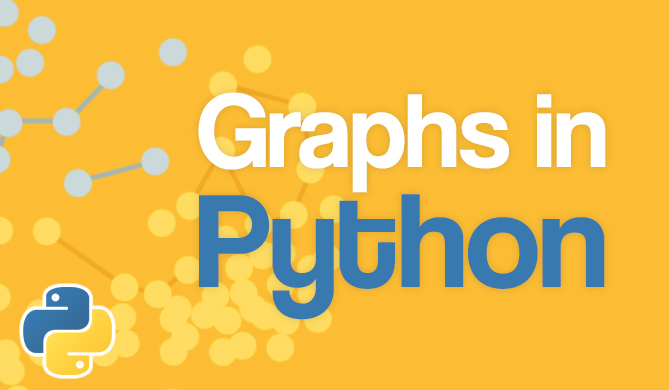Python: Check if Variable is a List

# Python: Check if Variable is a List### Introduction

Python is a dynamically typed language, and the variable data types are inferred without explicit intervention by the developer.

If we had code that needed a list but lacked type hints, which are optional, how can we avoid errors if the variable used is not a list?

In this tutorial, we'll take a look at how to check if a variable is a list in Python, using the type() and isinstance() functions, as well as the is operator:

Developers usually use type() and is, though, these can be limited in certain contexts, in which case, it's better to use the isinstance() function.

### Check if Variable is a List with type()

The built-in type() function can be used to return the data type of an object. Let's create a Dictionary, Tuple and List and use the type() function to check if a variable is a list or not:

grocery_list = ["milk", "cereal", "ice-cream"]
aTuple = ("apple", "banana", "cashew")

# Prints the type of each variable
print("The type of grocery_list is ", type(grocery_list))
print("The type of aTuple is ", type(aTuple))


This results in:

The type of grocery_list is  <class 'list'>
The type of aDict is  <class 'dict'>
The type of aTuple is  <class 'tuple'>


Now, to alter code flow programatically, based on the results of this function:

a_list = [1, 2, 3, 4, 5]

# Checks if the variable "a_list" is a list
if type(a_list) == list:
print("Variable is a list.")
else:
print("Variable is not a list.")


This results in:

"Variable is a list."


### Check if Variable is a List with is Operator

The is operator is used to compare identities in Python. That is to say, it's used to check if two objects refer to the same location in memory.

The result of type(variable) will always point to the same memory location as the class of that variable. So, if we compare the results of the type() function on our variable with the list class, it'll return True if our variable is a list.

Let's take a look at the is operator:

a_list = [1, 2, 3, 4, 5]
print(type(a_list) is list)


This results in:

True


Since this might look off to some, let's do a sanity check for this approach, and compare the IDs of the objects in memory as well:

print("Memory address of 'list' class:", id(list))


Now, these should return the same number:

Memory address of 'list' class: 4363151680


Note: You'll need to keep any subtypes in mind if you've opted for this approach. If you compare the type() result of any list sub-type, with the list class, it'll return False, even though the variable is-a list, although, a sub-class of it.

This shortfall of the is operator is fixed in the next approach - using the isinstance() function.

### Check if Variable is a List with isinstance()

The isinstance() function is another built-in function that allows you to check the data type of a variable. The function takes two arguments - the variable we're checking the type for, and the type we're looking for.

## Free eBook: Git Essentials

Check out our hands-on, practical guide to learning Git, with best-practices, industry-accepted standards, and included cheat sheet. Stop Googling Git commands and actually learn it!

This function also takes sub-classes into consideration, so any list sub-classes will also return True for being an instance of the list.

Let's try this out with a regular list and a UserList from the collections framework:

from collections import UserList

regular_list = [1, 2, 3, 4, 5]
user_list = [6, 7, 8, 9, 10]

# Checks if the variable "a_list" is a list
if isinstance(regular_list, list):
print("'regular_list' is a list.")
else:
print("'regular_list' is not a list.")

# Checks if the variable "a_string" is a list
if isinstance(user_list, list):
print("'user_list' is a list.")
else:
print("'user_list' is not a list.")


Running this code results in:

'regular_list' is a list.
'user_list' is a list.


### Conclusion

Python is a dynamically typed language, and sometimes, due to user-error, we might deal with an unexpected data type.

In this tutorial, we've gone over three ways to check if a variable is a list in Python - the type() function, the is operator and isinstance() function.

Last Updated: March 1st, 2021

Get tutorials, guides, and dev jobs in your inbox.Free
Course

### Graphs in Python - Theory and Implementation

# python# data structures# algorithms# computer science

Graphs are an extremely versatile data structure. More so than most people realize! Graphs can be used to model practically anything, given their nature of...DetailsProject

### Data Visualization in Python: The Collatz Conjecture

# python# matplotlib# data visualization

The Collatz Conjecture is a notorious conjecture in mathematics. A conjecture is a conclusion based on existing evidence - however, a conjecture cannot be proven....Details